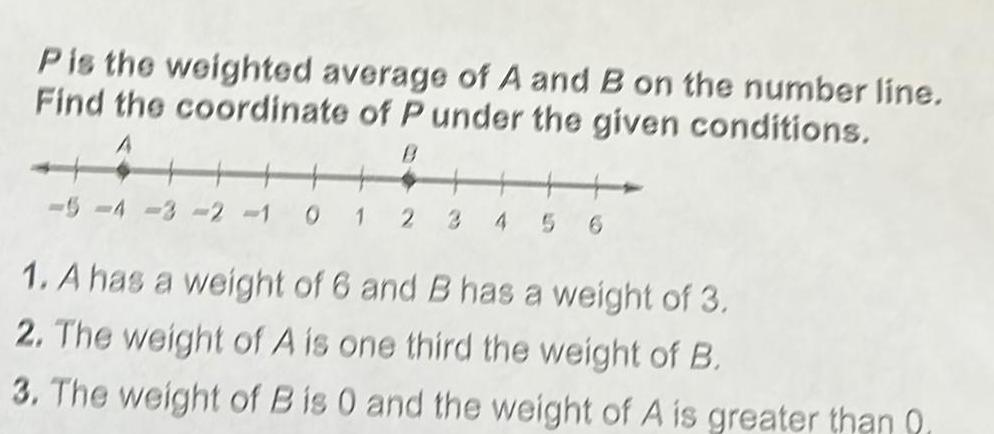Question:

# P is the weighted average of A and B on the number line Find

Last updated: 9/7/2023P is the weighted average of A and B on the number line Find the coordinate of P under the given conditions 5 4 3 2 1 0 1 2 4 1 A has a weight of 6 and B has a weight of 3 2 The weight of A is one third the weight of B 3 The weight of B is 0 and the weight of A is greater than 0